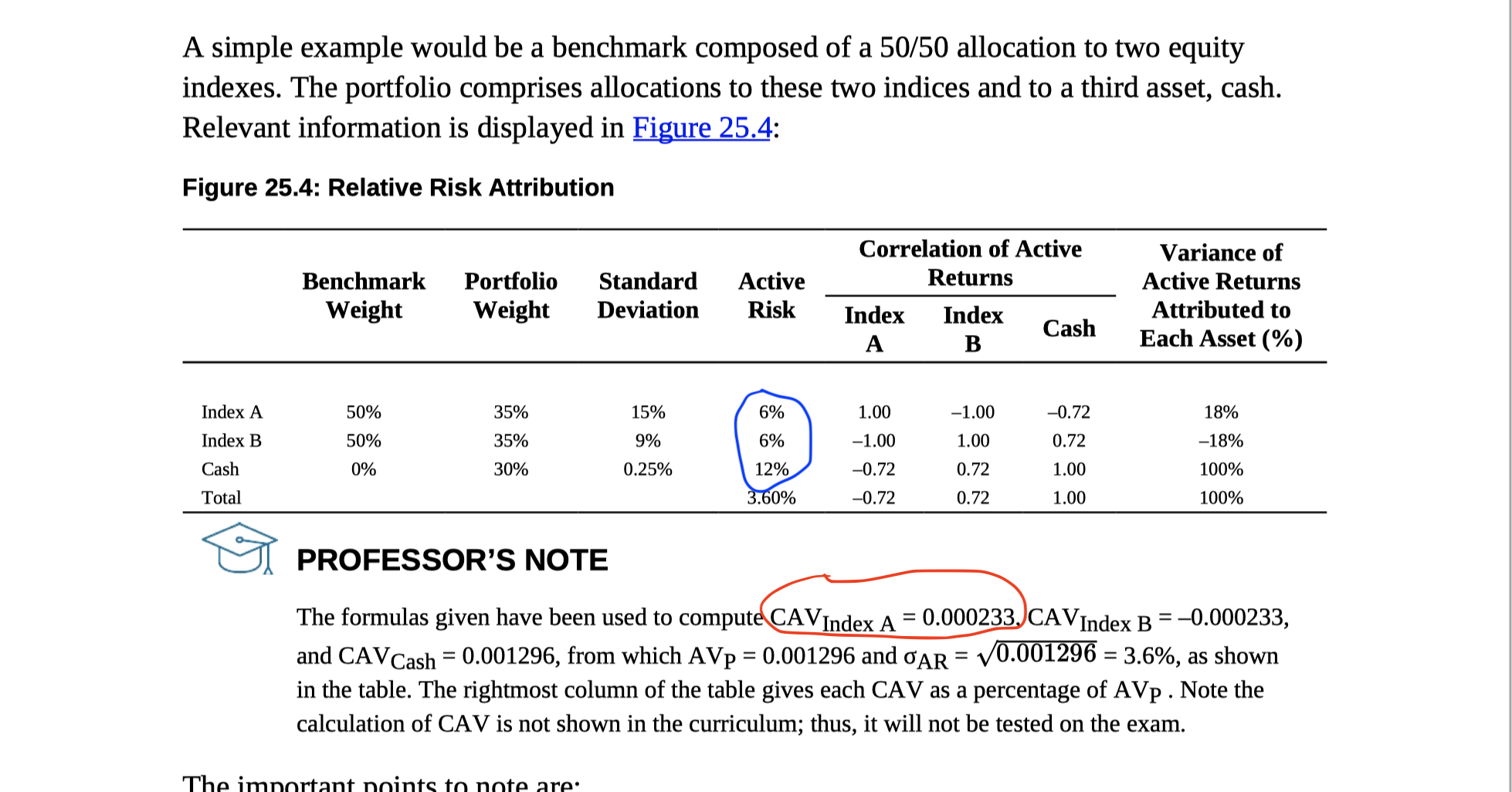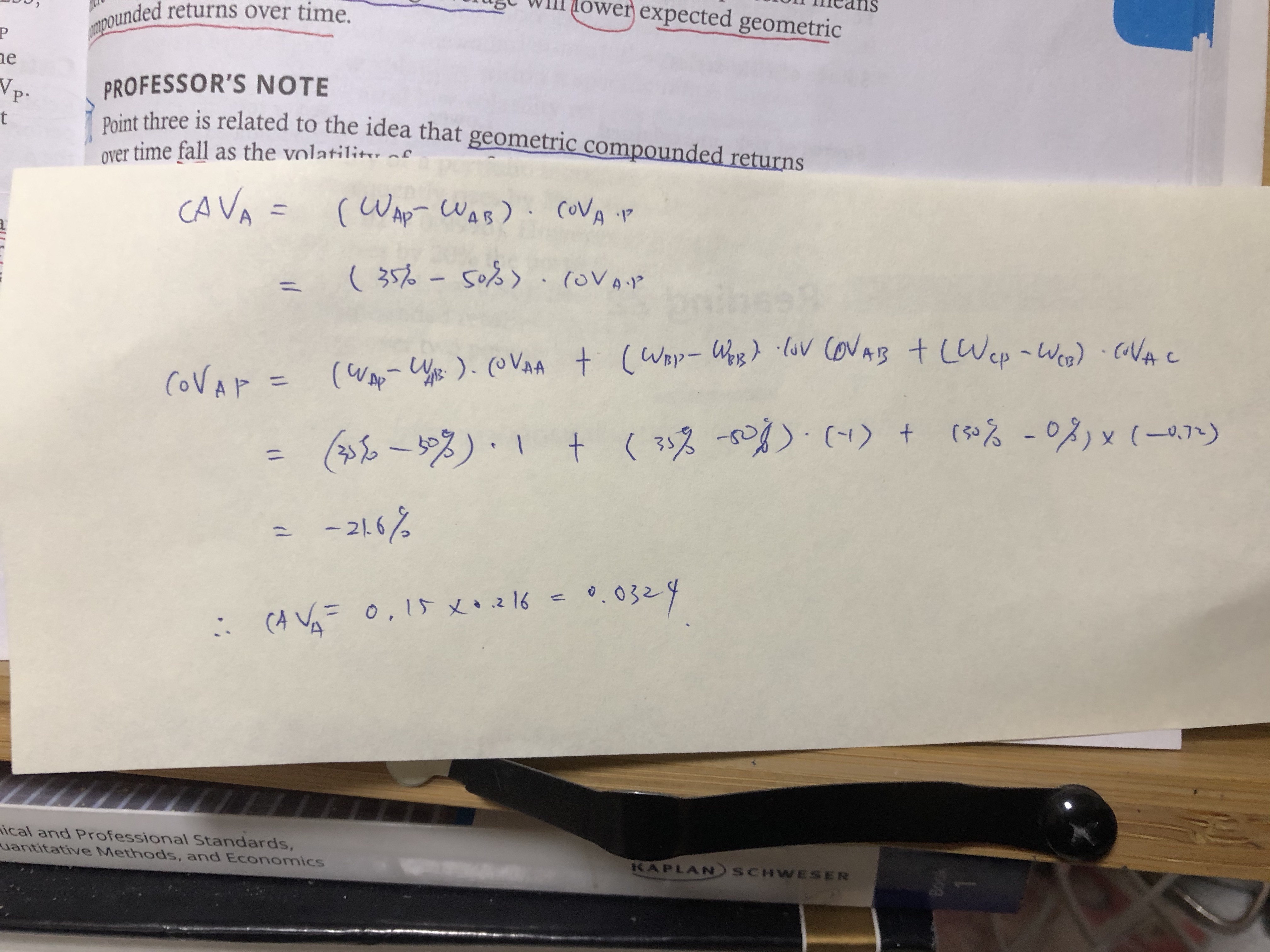# How to calculate VAV indexA?(this pic come from NOTEs book3 equity, page 198)

my question is:

1:How to calculate VAV indexA? the following process is my method, but the result is different ,what 's wrong?1. (the blue marked ) how to calculate the active risk?